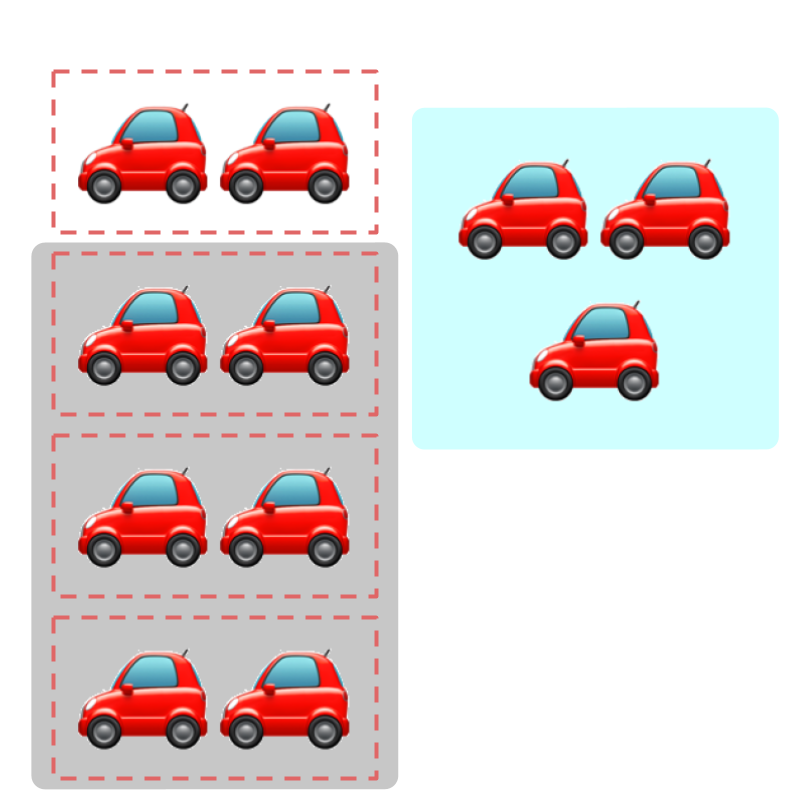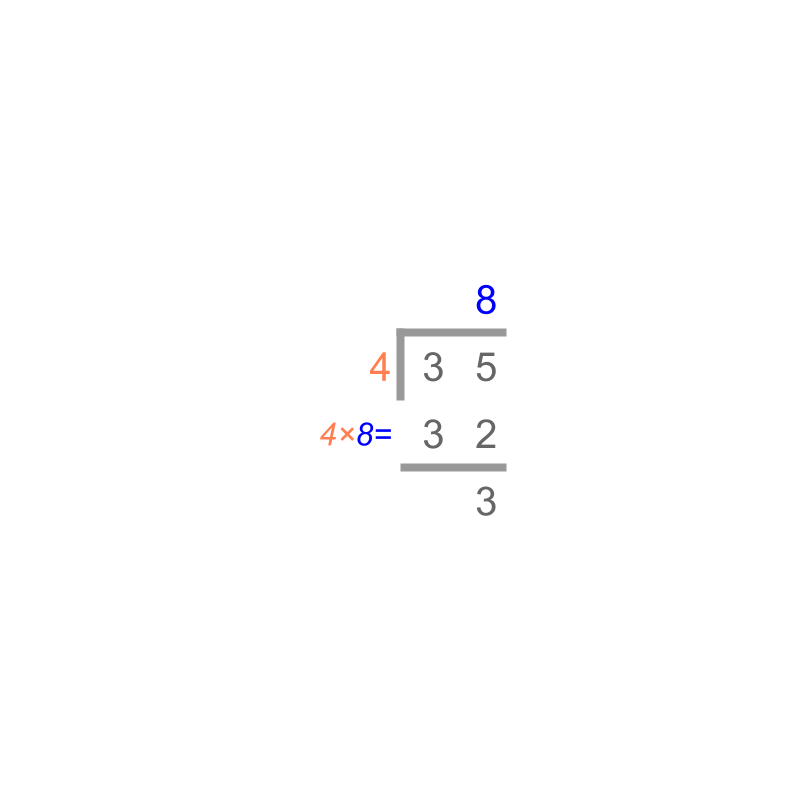maths > decimals

Decimal Division

what you'll learn...

overview

Decimals are fractions with standard place values. In this page, the following for decimals are explained.

•  Division in first principles -- splitting a quantity into a number of parts and measuring one part

•  simplified procedure : Sign property of division and long division method for Decimals.

recap

"Division" can be described as

dividend split into divisor number of parts.

$11÷4=$$11 \div 4 =$ $2$$2$ quotient and $3$$3$ remainder.Considering the figure with $11$$11$ cars and $4$$4$ dotted-boxes.$11$$11$ is split into $4$$4$ equal parts with each assigned $2$$2$ cars. There are $3$$3$ cars remaining. The remaining cars are not enough to assign equally to each dotted-box.

One part is counted as $2$$2$ and remaining amount is $3$$3$.

division is inverse of multiplication. And division of fractions are explained with that.

$\frac{3}{4}÷\frac{2}{3}$$\frac{3}{4} \div \frac{2}{3}$
$\quad = \frac{3}{4} \times \frac{3}{2}$ (multiply by inverse)
$\quad = \frac{9}{8}$.

splitting

Decimals are fractions with standardized place-values.

Consider $0.6÷3=0.2$$0.6 \div 3 = 0.2$.

By first principles, the dividend $0.6=\frac{6}{10}$$0.6 = \frac{6}{10}$ is split into divisor $3$$3$ number of equal parts : $\frac{2}{10};\frac{2}{10};\frac{2}{10}$. And one part is $\frac{2}{10}$$\frac{2}{10}$, or equals $0.2$$0.2$ in decimals.

Consider $0.6÷0.3=2$$0.6 \div 0.3 = 2$".

By first principles, the dividend $0.6=\frac{6}{10}$$0.6 = \frac{6}{10}$ is split into divisor $0.3=\frac{3}{10}$$0.3 = \frac{3}{10}$ number of equal parts :

This is done in two steps:
first step: $\frac{6}{10}$$\frac{6}{10}$ is split into $\frac{1}{10}$$\frac{1}{10}$ (denominator of $\frac{3}{10}$$\frac{3}{10}$) times is $\frac{60}{10}$$\frac{60}{10}$.
Second step: the result from the first step $\frac{60}{10}$$\frac{60}{10}$ is split into $3$$3$ (numerator of $\frac{3}{10}$$\frac{3}{10}$) times is $\frac{20}{10}$$\frac{20}{10}$

The result is $\frac{20}{10}$$\frac{20}{10}$, or equals $2$$2$ in decimals.

aligned or opposed to direction

Decimals are directed numbers, that is decimals are either positive or negative.

$0.2$$0.2$aligned in direction is "$+0.2$$+ 0.2$".

$0.2$$0.2$opposed in direction is "$-0.2$$- 0.2$".

Directed numbers, positive and negative, are explained as "aligned in direction" and "opposed in direction" respectively.

Consider $0.6÷\left(-0.3\right)=-2$$0.6 \div \left(- 0.3\right) = - 2$

By first principles, the dividend $0.6=\frac{6}{10}$$0.6 = \frac{6}{10}$aligned in direction is split into the divisor $0.3=\frac{3}{10}$$0.3 = \frac{3}{10}$opposed in direction number of equal parts :

This is done in two steps,
first step: $\frac{6}{10}$$\frac{6}{10}$aligned in direction is split into $\frac{1}{10}$$\frac{1}{10}$aligned in direction (denominator of $\frac{3}{10}$$\frac{3}{10}$) times is $\frac{60}{10}$$\frac{60}{10}$aligned in direction.
Second step: the result from the first step $\frac{60}{10}$$\frac{60}{10}$aligned in direction is split into $3$$3$ (numerator of $\frac{3}{10}$$\frac{3}{10}$)opposed in direction times is $\frac{20}{10}$$\frac{20}{10}$opposed in direction.

The result is $\frac{20}{10}$$\frac{20}{10}$opposed in direction, or equals $-2$$- 2$ in decimal number form.

decimal division

Decimal Division by first principle : Decimal division is splitting the dividend, into divisor number of parts with sign of the numbers (direction) handled appropriately.In whole numbers, we have studied Division by Place-value as illustrated in the figure. This procedure is used in decimals in a later step.

In Integers, we have studied Sign-property of Division.
•  +ve $÷$$\div$ +ve = +ve
•  +ve $÷$$\div$ -ve = -ve
•  -ve $÷$$\div$ +ve = -ve
•  -ve $÷$$\div$ -ve = +ve
This is applicable to decimals.

In Fractions, we have studied that division is inverse of multiplication.
For example, to divide $\frac{4}{5}÷\frac{3}{2}$$\frac{4}{5} \div \frac{3}{2}$, it is modified to $\frac{4}{5}×\frac{2}{3}$$\frac{4}{5} \times \frac{2}{3}$

Decimals are divided keeping in mind the place-value representation, which is a form of fractions.
For example, to divide $1.8÷.06$$1.8 \div .06$, it is equivalently thought as $\frac{18}{10}÷\frac{6}{100}$$\frac{18}{10} \div \frac{6}{100}$ and modified to multiplication $\frac{18}{10}×\frac{100}{6}$$\frac{18}{10} \times \frac{100}{6}$.

simplify

Consider division of $0.002÷0.05$$0.002 \div 0.05$

This is equivalently $\frac{2}{1000}÷\frac{5}{100}$$\frac{2}{1000} \div \frac{5}{100}$
$=\frac{2}{1000}×\frac{100}{5}$$= \frac{2}{1000} \times \frac{100}{5}$
$=\frac{2×100}{1000×5}$$= \frac{2 \times 100}{1000 \times 5}$
$=\frac{2}{50}$$= \frac{2}{50}$
$=0.04$$= 0.04$

Understanding the above, a simplified procedure to divide the decimals is devised.

The decimal point of dividend and divisor are removed and the numbers are divided as integers.
eg: $0.002$$0.002$ is modified to the integer form $2$$2$.
$0.05$$0.05$ is modified to the integer form $5$$5$.

The number of decimal-places in the dividend and divisor are counted.
eg: $0.002$$0.002$ has $3$$3$ decimal-places.
$0.05$$0.05$ has $2$$2$ decimal-places.

Now the integer forms are divided.
eg: $2÷5=0.4$$2 \div 5 = 0.4$

The number of decimal places of divisor is subtracted from dividend number of decimal places.
eg: $3-2=1$$3 - 2 = 1$.

The quotient form is modified to have the number of decimal points give by the difference above.
eg: $0.4$$0.4$ is modified to $0.04$$0.04$, that is decimal point moved to the left by $1$$1$.

example

Consider $1.55÷.005=310$$1.55 \div .005 = 310$".

$155÷5=31.$$155 \div 5 = 31.$

Difference in decimal places is $2-3=-1$$2 - 3 = - 1$

So the product decimal place moves $1$$1$ place to the right.
$1.55÷0.005=310$$1.55 \div 0.005 = 310$

summary

Decimal Division -- Simplified Procedure :
The signs (+ve / -ve) are handled as in Sign-property of Integer Division
•  +ve $÷$$\div$ +ve = +ve
•  +ve $÷$$\div$ -ve = -ve
•  -ve $÷$$\div$ +ve = -ve
•  -ve $÷$$\div$ -ve = +ve

The decimal places are removed and the division is carried out as per Whole number Division by Place Value.

•  The decimal-point is modified in the result
•  The decimal-point is moved to the left the number of times equal to the difference number of decimals in dividend minus number of decimals in divisor. A positive difference moves the decimal point to the left, and a negative difference moves the decimal point to the right.

Outline